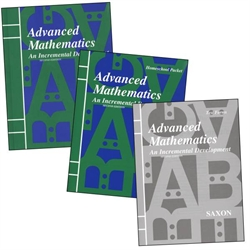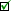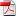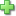# Saxon Advanced Mathematics - Home Study Kit

Publisher: Saxon Publishers
Curriculum Bundle, 159 pages
Price: \$160.90
Used Price: \$78.00 (2 in stock)Condition Policy

See series description for full review.

In Advanced Mathematics, topics from advanced algebra, geometry, trigonometry, discrete mathematics, and mathematical analysis are interwoven to form a fully integrated text. Specific topics include permutations and combinations; trigonometric identities; inverse trigonometric functions; conic sections; graphs of sinusoids; rectangular and polar representation of complex numbers; De Movie's theorem; matrices and determinants; the binomial theorem; and the rational roots theorem. Euclidean geometry is also included. (125 lessons)

The student edition contains answers to odd-numbered problems; the Home Study Packet, which is the complete answer key and tests, is also included. You will need a scientific calculator, but since there are optional exercises for a graphing calculator, that would be even better.

## Samples:Lesson 2 page 1Lesson 2 page 2Test 7Answer Key

Did you find this review helpful?
Series Description
Related CategoriesClick here to write a review